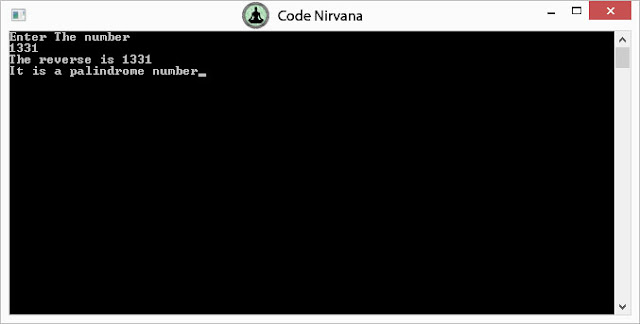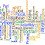# Program to check if a number is palindrome (reverse a number) in C++

Reverse a number using the function reverse() given below. the execution of following code will tell you if the input number is palindrome (Number / text which when reversed is the same)

```/* Palindrome numbers program

#include<iostream>

#include<conio.h>

using namespace std;

int reverse(int);

int main()

{

int num;

cout<<"Enter The number"<<endl;

cin>>num;

cout<<"The reverse is "<<reverse(num)<<endl;;

if(reverse(num)==num) cout<<"It is a palindrome number";

else cout<<"Not a palindrome number";

getch();

return 0;

}

int reverse(int a)

{

int temp=0;

for(;a!=0;a/=10)

{

temp=temp*10+(a%10);

}

return temp;

}

```

OUTPUT :i want program for pattern:
1
2 3
6 5 4
7 8 9 10
15 14 13 12 11
if i give input as n=5i want program for pattern:
1
2 3
6 5 4
7 8 9 10
15 14 13 12 11
if i give input as n=5what is the significance or meaning of +a+ and +a